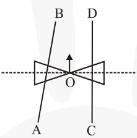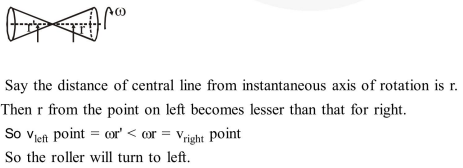# A roller is made by joining

Question:

A roller is made by joining together two cones at their vertices $\mathrm{O}$. It is kept on two rails AB and CD which are placed asymmetrically (see figure), with its axis perpendicular to CD and its centre $\mathrm{O}$ at the centre of line joining $\mathrm{AB}$ and $\mathrm{CD}$ (see figure). It is given a light push so that it starts rolling with its centre $\mathrm{O}$ moving parallel to $\mathrm{CD}$ in the direction shown. As it moves, the roller will tend to :-1. turn left and right alternately.

2. turn left.

3. turn right.

4. go straight.

Correct Option: , 2

Solution: